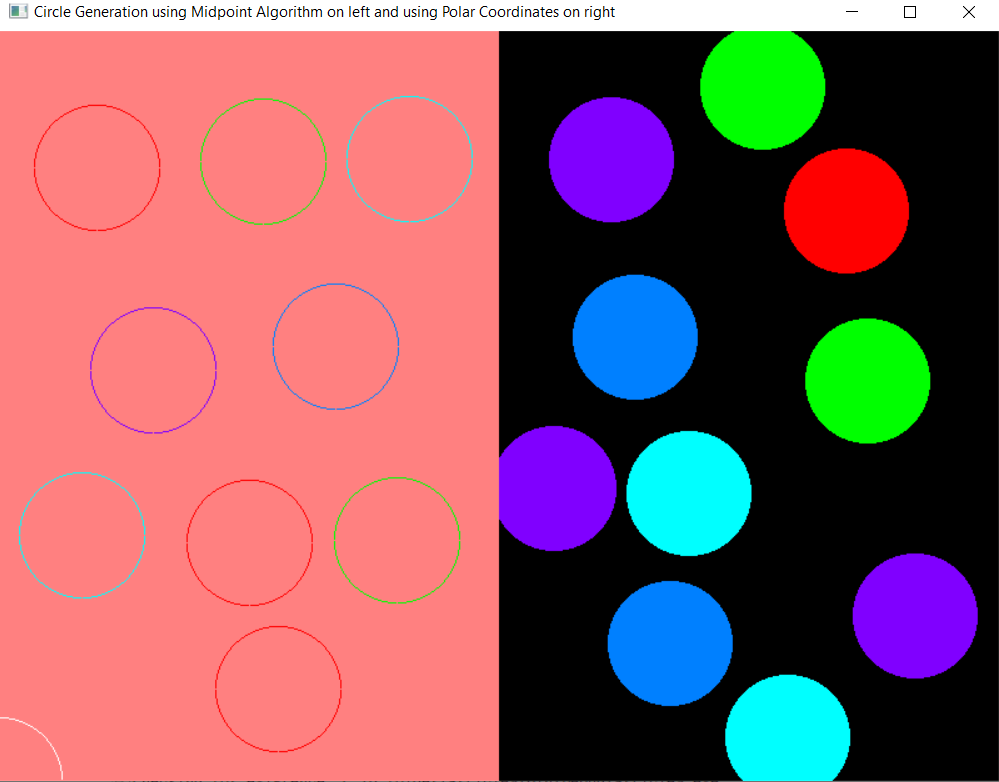# Draw circles using the polar coordinates and mid-point circle drawing algorithm on the same console using openGL in C++

• Last Updated : 16 Jul, 2021

In this article, the task is to draw circles in two different subwindows using two different algorithms, and the circles are created using a single mouse click.

Approach:

• There are two subwindows and both of them use different algorithms to create circles.
• The left subwindow creates a circle using Midpoint Circle drawing algorithm and the right subwindow implements the concept of polar coordinates.
• A circle can be created anywhere on the console using a single left mouse click and the coordinates of the center of the circle created depends on the position of the click.
• To change the color of the circle, right-click on the mouse.
• After performing all operations, jump out of the program by simply pressing the Esc key on the keyboard.

Below is the implementation of the above approach:

## C++

 `// C++ program to implement onClick` `// functionality in OpenGL to draw` `// a circle using polar coordinates` `// and midpoint algorithm` `#include ` `#include ` `#include ` `#include ` `#define xpix 500` `#include ` `using` `namespace` `std;`   `float` `r, g, b, x, y;` `bool` `flag = ``true``;` `int` `counter = 0;`   `// Function to plot the coordinates` `void` `plot(``int` `x1, ``int` `y1)` `{` `    ``// Initialize the points` `    ``glBegin(GL_POINTS);`   `    ``// Draw the vertex` `    ``glVertex2i(x1 + x, y1 + y);`   `    ``// End` `    ``glEnd();` `}`   `// Function works on mouse click` `void` `mouse(``int` `button, ``int` `state,` `           ``int` `mousex, ``int` `mousey)` `{` `    ``// Check if the current click` `    ``// is left-click or not` `    ``if` `(button == GLUT_LEFT_BUTTON` `        ``&& state == GLUT_DOWN) {` `        ``flag = ``true``;` `        ``x = mousex;` `        ``y = 600 - mousey;` `    ``}`   `    ``// Check if the current click` `    ``// is right-click or not` `    ``else` `if` `(button == GLUT_RIGHT_BUTTON` `             ``&& state == GLUT_DOWN) {` `        ``if` `(counter > 4) {` `            ``counter = 0;` `        ``}`   `        ``counter++;`   `        ``// Redisplay` `        ``glutPostRedisplay();` `    ``}` `}`   `// Function that exits from program` `void` `keyboard(unsigned ``char` `key,` `              ``int` `x, ``int` `y)` `{` `    ``switch` `(key) {` `    ``case` `27:` `        ``glutHideWindow();` `    ``}` `}`   `// Function to draw the circles using` `// the midpoint circle algorithms` `void` `midPointCircleDraw(``void``)` `{` `    ``// Change the color of circles on` `    ``// the right click` `    ``if` `(counter == 1) {` `        ``glColor3f(1, 0, 0);` `    ``}` `    ``else` `if` `(counter == 2) {` `        ``glColor3f(0, 1, 0);` `    ``}` `    ``else` `if` `(counter == 3) {` `        ``glColor3f(0, 1, 1);` `    ``}` `    ``else` `if` `(counter == 4) {` `        ``glColor3f(0.5, 0, 1);` `    ``}` `    ``else` `if` `(counter == 5) {`   `        ``glColor3f(0, 0.5, 1);` `    ``}`   `    ``// Matrix mode` `    ``glMatrixMode(GL_PROJECTION);` `    ``glLoadIdentity();`   `    ``// Given the coordinates` `    ``gluOrtho2D(0.0, 400.0, 0.0, 600.0);` `    ``int` `r = 50;`   `    ``if` `(flag) {`   `        ``// Begin the pointer` `        ``glBegin(GL_POLYGON);` `        ``int` `x1 = 0;` `        ``int` `y1 = r;`   `        ``// Initialising the value` `        ``// of the decision` `        ``float` `decision = 5 / 4 - r;`   `        ``// Print the initial point on` `        ``// the axes after translation` `        ``plot(x1, y1);`   `        ``while` `(y1 > x1) {`   `            ``// Mid-point is inside or` `            ``// on the perimeter` `            ``if` `(decision < 0) {` `                ``x1++;` `                ``decision += 2 * x1 + 1;` `            ``}`   `            ``// Mid-point is outside` `            ``// the perimeter` `            ``else` `{` `                ``y1--;` `                ``x1++;` `                ``decision += 2 * (x1 - y1) + 1;` `            ``}`   `            ``// Plot the points in the` `            ``// different octants` `            ``plot(x1, y1);` `            ``plot(x1, -y1);` `            ``plot(-x1, y1);` `            ``plot(-x1, -y1);` `            ``plot(y1, x1);` `            ``plot(-y1, x1);` `            ``plot(y1, -x1);` `            ``plot(-y1, -x1);` `        ``}` `    ``}`   `    ``glFlush();` `}`   `// Function to draw the circle using` `// the polar coordinates` `void` `polarCoordinateCircleDraw(``void``)` `{` `    ``float` `angle_theta;`   `    ``// Change the color of the circles` `    ``// on right click` `    ``if` `(counter == 1) {` `        ``glColor3f(1, 0, 0);` `    ``}` `    ``else` `if` `(counter == 2) {` `        ``glColor3f(0, 1, 0);` `    ``}` `    ``else` `if` `(counter == 3) {` `        ``glColor3f(0, 1, 1);` `    ``}` `    ``else` `if` `(counter == 4) {` `        ``glColor3f(0.5, 0, 1);` `    ``}` `    ``else` `if` `(counter == 5) {`   `        ``glColor3f(0, 0.5, 1);` `    ``}`   `    ``// Matrix mode` `    ``glMatrixMode(GL_PROJECTION);` `    ``glLoadIdentity();`   `    ``// Given the coordinates` `    ``gluOrtho2D(0.0, 800.0, 0.0, 600.0);` `    ``if` `(flag) {`   `        ``// Begin the pointer` `        ``glBegin(GL_POLYGON);`   `        ``// Iterate through all the` `        ``// 360 degrees` `        ``for` `(``int` `i = 0; i < 360; i++) {`   `            ``angle_theta = i * 3.142 / 180;` `            ``glVertex2f(x + 50 * ``cos``(angle_theta),` `                       ``y + 50 * ``sin``(angle_theta));` `        ``}`   `        ``// Set the vertex` `        ``glEnd();` `    ``}`   `    ``// Flushes the frame buffer to` `    ``// the screen` `    ``glFlush();` `}`   `// Driver Code` `int` `main(``int` `argc, ``char``** argv)` `{` `    ``// Initialize the drivers` `    ``glutInit(&argc, argv);`   `    ``// Initialize the display mode` `    ``glutInitDisplayMode(` `        ``GLUT_SINGLE | GLUT_RGB);`   `    ``// Update the window size` `    ``glutInitWindowSize(800, 600);`   `    ``// Update the window position` `    ``glutInitWindowPosition(100, 100);`   `    ``int` `mainWindow = glutCreateWindow(` `        ``"Circle Generation using "` `        ``"Midpoint Algorithm on "` `        ``"left and using Polar "` `        ``"Coordinates on right"``);`   `    ``// Set the background color of` `    ``// the right sub-window` `    ``glClearColor(0, 0, 0, 0);`   `    ``// Clears the frame buffer` `    ``glClear(GL_COLOR_BUFFER_BIT);`   `    ``// Links display event with the` `    ``// display the event handler` `    ``// (polarCoordinateCircleDraw)` `    ``glutDisplayFunc(` `        ``polarCoordinateCircleDraw);`   `    ``// Mouse event handler` `    ``glutMouseFunc(mouse);`   `    ``// Create the sub-window to implement` `    ``// midpoint algorithm on left` `    ``int` `subWindow1` `        ``= glutCreateSubWindow(` `            ``mainWindow, 0, 0, 400, 600);`   `    ``glutInitWindowPosition(100, 100);`   `    ``// Sets the background color of` `    ``// the left sub-window` `    ``glClearColor(1, 0.5, 0.5, 1);`   `    ``// Clears the frame buffer` `    ``glClear(GL_COLOR_BUFFER_BIT);`   `    ``// Links display event with the` `    ``// display the event handler` `    ``// (midPointCircleDraw)` `    ``glutDisplayFunc(midPointCircleDraw);`   `    ``// Mouse event handler` `    ``glutMouseFunc(mouse);`   `    ``// Keyboard event handler` `    ``glutKeyboardFunc(keyboard);`   `    ``// Loops the current event` `    ``glutMainLoop();` `}`

Output:My Personal Notes arrow_drop_up
Recommended Articles
Page :